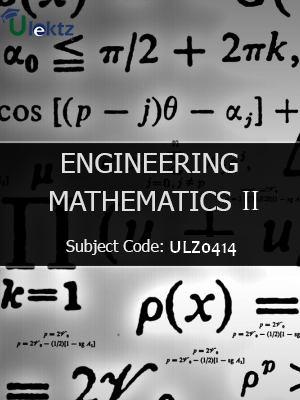•My WalletMy Order
•My Profile
•My Connections
•My Books
•My Videos
•My Tests
•My Calender
•My Messages
•My Shopping Cart
•My Orders
•Account Settings
•Help

# Book Details# Engineering Mathematics-II

 Course Code : ULZ0414 Author : uLektz University : General for All University Regulation : 2017 Categories : Electronics & Communication Format :ePUB3 (DRM Protected) Type : eBook

FREE

Description :Engineering Mathematics-II of ULZ0414 covers the latest syllabus prescribed by General for All University for regulation 2017. Author: uLektz, Published by uLektz Learning Solutions Private Limited.

Note : No printed book. Only ebook. Access eBook using uLektz apps for Android, iOS and Windows Desktop PC.

##### Topics
###### UNIT I MATRICES

1.1 Eigenvalues and Eigenvectors of a real matrix

1.2 Characteristic equation

1.3 Properties of Eigen values and Eigenvectors

1.4 Cayley - Hamilton theorem

1.5 Diagonalization of matrices

1.6 Reduction of a quadratic form to canonical form by orthogonal transformation

###### UNIT II VECTOR CALCULUS

2.1 Gradient and directional derivative - Divergence and curl

2.2 Vector identities -Irrotational and Solenoidal vector fields

2.3 Line integral over a plane curve - Surface integral - Area of a curved surface - Volume integral

2.4 Green’s theorem - Gauss divergence

2.5 Stoke’s theorems

2.6 Verification and application in evaluating line, surface and volume integrals

###### UNIT III ANALYTIC FUNCTIONS

3.1 Analytic function - Necessary and sufficient conditions for analyticity in Cartesian and polar coordinates - Properties

3.2 Harmonic conjugates

3.3 Construction of analytic function

3.4 Conformal mapping - Mapping by functions w = z + c, cz, 1/z,z2

3.5 Bilinear transformation

###### UNIT IV COMPLEX INTEGRATION

4.1 Line integral

4.2 Cauchy’s integral theorem, Cauchy’s integral formula

4.3 Taylor’s and Laurent’s series

4.4 Singularities

4.5 Residues - Residue theorem

4.6 Application of residue theorem for evaluation of real integrals - Use of circular contour and semicircular contour

###### UNIT V LAPLACE TRANSFORMS

5.1 Existence conditions - Transforms of elementary functions - Transform of unit step function and unit impulse function - Basic properties

5.2 Shifting theorems

5.3 Transforms of derivatives and integrals

5.4 Initial and final value theorems, Inverse transforms - Convolution theorem

5.5 Transform of periodic functions

5.6 Application to solution of linear second order ordinary differential equations with constant coefficients